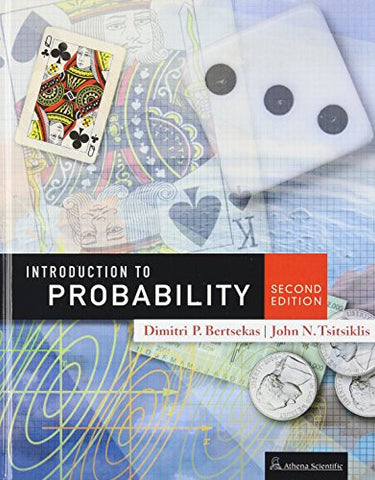# Introduction to Probability, 2nd Edition

• \$98.83
• Save \$28
• Binding: Hardcover
• Author: Dimitri P. Bertsekas;John N. Tsitsiklis
• Publish Date: 2008-07-15

Attention : For textbook, access codes and supplements are not guaranteed with used items.

An intuitive, yet precise introduction to probability theory, stochastic processes, and probabilistic models used in science, engineering, economics, and related fields. The 2nd edition is a substantial revision of the 1st edition, involving a reorganization of old material and the addition of new material. The length of the book has increased by about 25 percent. The main new feature of the 2nd edition is thorough introduction to Bayesian and classical statistics.

The book is the currently used textbook for Probabilistic Systems Analysis, an introductory probability course at the Massachusetts Institute of Technology, attended by a large number of undergraduate and graduate students. The book covers the fundamentals of probability theory (probabilistic models, discrete and continuous random variables, multiple random variables, and limit theorems), which are typically part of a first course on the subject, as well as the fundamental concepts and methods of statistical inference, both Bayesian and classical. It also contains, a number of more advanced topics, from which an instructor can choose to match the goals of a particular course. These topics include transforms, sums of random variables, a fairly detailed introduction to Bernoulli, Poisson, and Markov processes.

The book strikes a balance between simplicity in exposition and sophistication in analytical reasoning. Some of the more mathematically rigorous analysis has been just intuitively explained in the text, but is developed in detail (at the level of advanced calculus) in the numerous solved theoretical problems.

Written by two professors of the Department of Electrical Engineering and Computer Science at the Massachusetts Institute of Technology, and members of the prestigious US National Academy of Engineering, the book has been widely adopted for classroom use in introductory probability courses within the USA and abroad.

From a Review of the 1st Edition:

...it trains the intuition to acquire probabilistic feeling. This book explains every single concept it enunciates. This is its main strength, deep explanation, and not just examples that happen to explain. Bertsekas and Tsitsiklis leave nothing to chance. The probability to misinterpret a concept or not understand it is just... zero. Numerous examples, figures, and end-of-chapter problems strengthen the understanding. Also of invaluable help is the book's web site, where solutions to the problems can be found-as well as much more information pertaining to probability, and also more problem sets. --Vladimir Botchev, Analog Dialogue

Several other reviews can be found in the listing of the first edition of this book. Contents, preface, and more info at publisher's website (Athena Scientific, athenasc com)